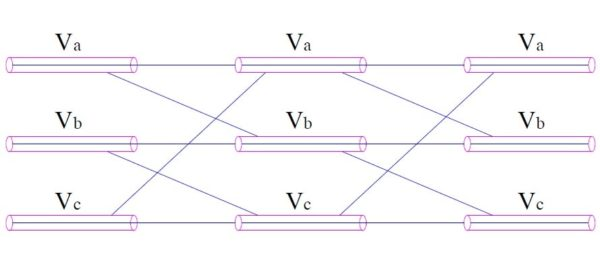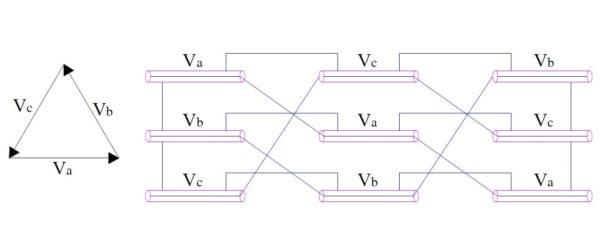# Why Cable Bonding Required?

Cable bonding is basically the method of making electrical connection between the sheaths of two cables. Such electrical connection of sheaths may either be done at one end or at both the ends of the cable depending upon the application. In this post we will discuss the importance of bonding of cable.

As we know that a pulsating magnetic field is set up around ac current carrying conductor, therefore a cable carrying current I will also create pulsating magnetic flux. This magnetic flux will link with the sheath of the cable and hence will generate an induced voltage. If the cable sheath of two single core cable system is bonded at both the ends then the induced voltage will give rise to a circulating current. This circulating current (known as sheath circuit current) will complete its circuit through the sheath of one cable to another and will contribute to sheath losses. But if the cable sheaths are not bonded then the induced voltage in the sheath will not cause to flow sheath circulating current. So you may think that cable bonding is not desired. But it is not so. Why? You will get the answer after reading the full content.

Let us now find the value of induced voltage in sheath of two single core cable system. Let the induced voltage be V.

Therefore V = ωMI where M is the mutual inductance between the core of cable and its sheath.

Mutual Inductance M between the core and sheath of cable is defined as the flux linking both the core and sheath due to per ampere current flow in the core. This mutual inductance is given as

M = 2×10-7ln(d/rm) H / meter

where d = distance between the centre of two cables

rm = GMR of cable

The above calculation of mutual inductance M of two single core cable is done in the same manner as calculated for overhead lines. Please refer Geometrical Mean Radius (GMR) and Inductance for understanding the calculation.

Therefore the voltage induced across the sheath at the far end from point of bonding will be

V = 2ωIx10-7ln(d/rm) V / meter

If the sheaths of the two cables are bonded at a single point, then the voltage across the sheaths of the two cable will be

2V = 4ωIx10-7ln(d/rm) V / meter

In case of short circuit, the current I will be of large magnitude which will lead to higher voltage across the sheaths. In case the cable sheaths are not bonded at both ends, this higher voltage will cause sparking and subsequent pitting and damage of cable sheaths. Thus it is very important to have bonding of cable sheaths.

Now you might think that how do we reduce the losses in sheaths which results due to sheath circulating current due to bonding? Well, to reduce the sheath loss, what we can do is to minimize the sheath circulating current. The sheath circulating current can be minimized by minimizing the induced voltage. But induced voltage can be minimized by minimizing the inductance of individual cable. Thus our aim is to minimize the inductance of cable to minimize sheath circulating current and hence sheath loss.

To minimize the inductance of cables in 3 phase system, method of cable cross-bonding as shown in figure below is employed.Since the three phase voltage are 120 degree apart and equal in magnitude, the net induced sheath voltage will sum up to zero if the cables are placed symmetrically along the vertices of equilateral triangle. But there always exists asymmetry, this means that the net voltage will not be zero but some voltage will exist. This non-zero voltage may drive appreciable amount of sheath circulating current and hence sheath loss.

Therefore to eliminate the residual sheath voltage completely, the best way is transpose the cables at a regular interval as in overhead lines along with cross-bonding.This method is very effective in eliminating the residual sheath voltage and hence sheath circulating current. Thus sheath loss due to bonding is eliminated.

This site uses Akismet to reduce spam. Learn how your comment data is processed.